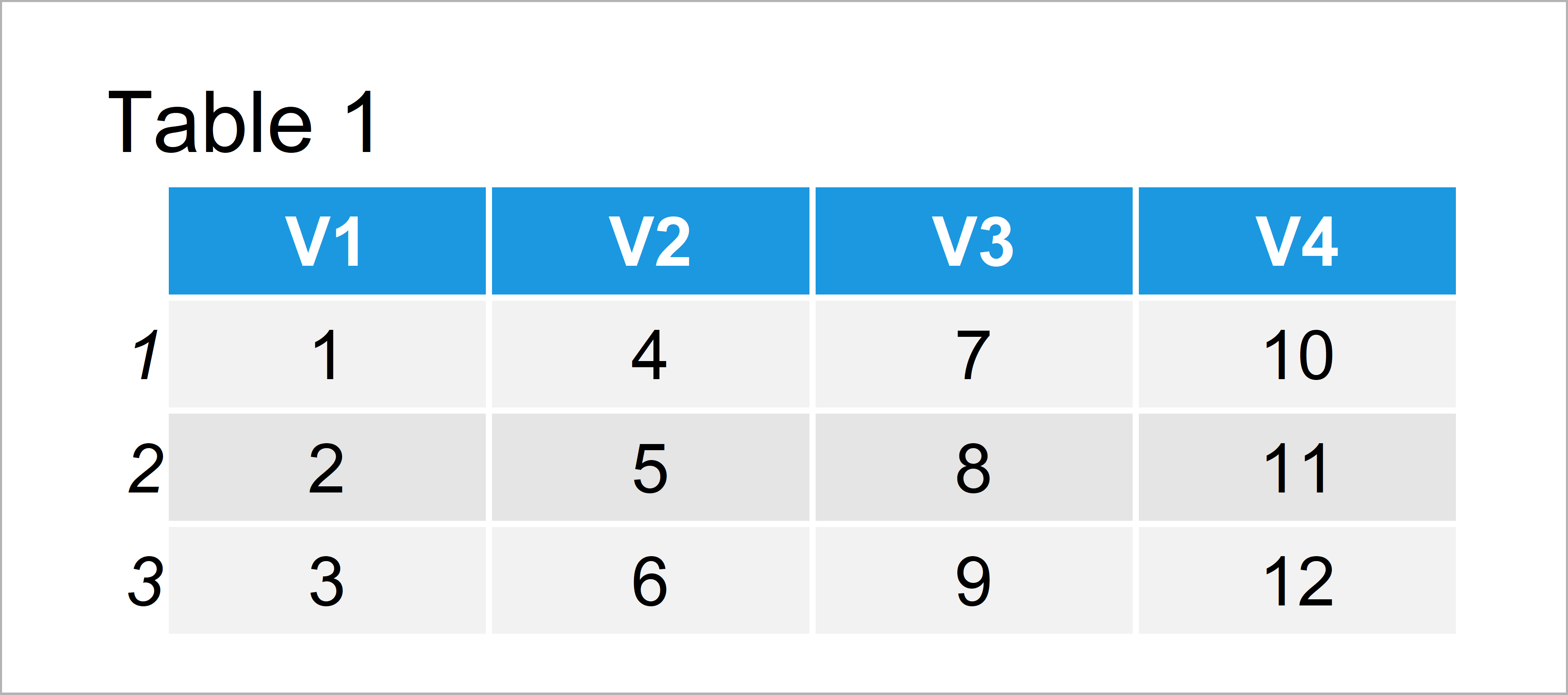# Select Subset of Data Table Columns in R (Example)

In this article, I’ll illustrate how to extract certain variables from a data.table in R.

Let’s take a look at some R codes in action:

## Example Data & Add-On Packages

To be able to use the functions of the data.table package, we have to install and load data.table first:

```install.packages("data.table")                            # Install data.table package

We use the following data.table as basement for this R tutorial:

```dat_tab <- data.table(matrix(1:12, ncol = 4))             # Create example data table
dat_tab                                                   # Print example data table```As you can see based on Table 1, our exemplifying data is a data.table containing three rows and four variables.

## Example: Remove Columns from Data Table Using with = FALSE

This example illustrates how to drop a specific set of variables from our data.table.

For this, we have to specify a vector of column names that we want to retain. Furthermore, we have to set the with argument to be equal to FALSE:

```dat_tab_new <- dat_tab[ , ! c("V1", "V3"), with = FALSE]  # Remove columns
dat_tab_new                                               # Print updated data table```In Table 2 you can see that we have constructed a data.table containing only two columns by running the previous code.

## Video, Further Resources & Summary

Some time ago I have published a video on my YouTube channel, which illustrates the R programming codes of this tutorial. You can find the video below.

Also, you might have a look at some of the related R articles of this website:

In summary: In this article you have learned how to retain particular columns of a data.table in the R programming language. If you have additional questions, don’t hesitate to let me know in the comments.

Subscribe to the Statistics Globe Newsletter